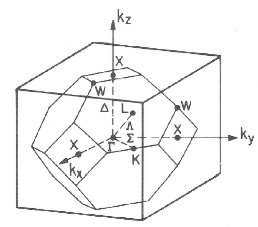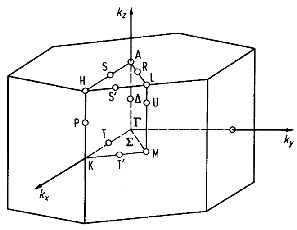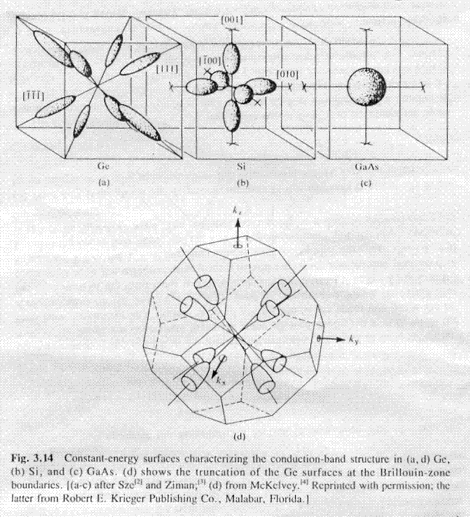nextnano.com  GUI: nextnanomat  Tool: nextnano++  Tool: nextnano³  Tool: nextnano.NEGF  Download | Search | Copyright | News | Publications  * password protected nextnano³ softwareEffective masses

# Database

## Effective masses

• ``` conduction-band-masses    = m    m    m       ! ```Gamma valley (m,m,m)```                              ml,L  mt,L  mt,L    ! ```L valley  (ml,mt,mt)```                              ml,X  mt,X  mt,X    ! ```X valley  (ml,mt,mt)
`1/m ` are the masses in the principal axes system of the minima. These masses are associated to the eigenvectors of the minima in the order they are given in the parameter set. The eigenvectors are specified by their coordinates in the cartesian coordinate system of the crystal.
• `valence-band-masses`

## Conduction band masses

We have to specify 9 values for the conduction band masses. For each valley (Gamma, L, X) we have one line and for each valley we need to specify the longitudinal (ml) and the transverse masses (mt1,mt2) where mt1=mt2=mt.

e.g. GaAs

```                             1st      2nd      3rd principal axis```

``` conduction-band-masses    = 0.067d0  0.067d0  0.067d0  ! ```Gamma valley (m,m,m)```                              1.9d0    0.075d0  0.075d0  ! ```L valley  (ml,mt,mt)```                              1.98d0   0.27d0   0.27d0   ! ```X valley  (ml,mt,mt)```                             ```  unit:  [m0]  (free electron mass)

The order is important for the L and X valleys (mlongitudinal, mtransverse, mtransverse).

``` conduction-band-masses    = m    m    m       ! ```Gamma valley (m,m,m)```                              ml,L  mt,L  mt,L    ! ```L valley  (ml,mt,mt)```                              ml,X  mt,X  mt,X    ! ```X valley  (ml,mt,mt)```  ```

These masses represent a mass tensor (1/m)ij given in the principal-axes system:

```  1/m     0      0    ! ```Gamma valley```    0     1/m     0    !    0      0     1/m   !  ```

```  1/ml,L   0      0    ! ```L valley```    0     1/mt,L   0    !    0      0     1/mt,L !  ```

```  1/ml,X   0      0    ! ```X valley```    0     1/mt,X   0    !    0      0     1/mt,X !  ```This picture shows the first Brillouin zone for zinc blende and diamond structure crystals (left) and for wurtzite (right).Constant-energy surfaces characterizing the conduction-band-structure in (a,d) Ge, (b) Si, and (c) GaAs. (d) shows the truncation of the Ge surfaces at the Brillouin-zone boundaries.

The degeneracy of the Gamma valley is 1, of the L valley it is 8 and of the X valley it is 6. The L and X valleys are shared with the adjacent first Brillouin zone thus we take only half the value:

`conduction-band-degeneracies = 2 8 6  ! `(including spin degeneracy)``` number-of-minima-of-cband    = 1 4 3  ```

In the case of Si, where the X valleys lie completely inside the first Brillouin zone, we take twice as much as the conduction band degeneracy for its valleys. The degeneracy includes the number of degenerate minima per band as well as the twofold spin-degeneracy.

`conduction-band-degeneracies = 2 8 12  ! `(including spin degeneracy)``` number-of-minima-of-cband    = 1 4 6   ! ```Number of minima (without spin degeneracy) in each set of degenerate minima.

Now we have to specify, where these minima are located in the crystal-axes system (components of k vector along crystal xyz [k0]). The position of the minima in k-space is defined by the specifier `conduction-band-minima`. The coordinates are in units of``` [2pi/a] ```within the crystal coordinate system where` a `is the lattice constant.
Note: Currently it is assumed in parts of the program, that the ordering of the conduction band minima is like
1=Gamma (zone center), 2=L (along <111> direction), 3=X (along <100> direction).

`conduction-band-minima   = v11 v12 v13`
```                           v21 v22 v23```
```                           v31 v32 v33```
```                           ...```
k vectors to individual conduction band minima.
As many vectors (coordinate triplets in crystal coordinate system) as individual minima.
Let's assume we have 3 conduction band minima 1,2,3 as specified above.
These minima are` deg1,deg2,deg`3-fold degenerate. In this case, input for` deg1/2+deg2/2+deg3/2 ` vectors has to be provided. The factor 1/2 is due to spin degeneracy which is already included in the degeneracy factors.

GaAs:

``` conduction-band-minima =     0d0      0d0      0d0     ! Gamma                                                         !                               0.866d0  0.866d0  0.866d0 ! L1  1 1 1                               0.866d0  0.866d0 -0.866d0 ! L2  1 1-1                              -0.866d0  0.866d0  0.866d0 ! L3 -1 1 1                              -0.866d0  0.866d0 -0.866d0 ! L4 -1 1-1                                                         !                               1d0      0d0      0d0     ! X1  1 0 0                               0d0      1d0      0d0     ! X2  0 1 0                               0d0      0d0      1d0     ! X3  0 0 1```

(We have to specify only half the amount of the L and X minima (namely 4 instead of 8 (L) and 3 instead of 6 (X)). For Si we have to specify 6 X valleys as they lie completely inside the first Brillouin zone.
(The value of 0.866 is due to 31/2/2 = 0.866025.)

Si: (X valley minimum at e.g. at k=(0.85,0,0) ``` [2pi/a] ```where` a `is the lattice constant.)

``` conduction-band-minima =     0d0      0d0      0d0     ! Gamma                                                         !                               0.866d0  0.866d0  0.866d0 ! L1   1 1 1                               0.866d0  0.866d0 -0.866d0 ! L2   1 1-1                              -0.866d0  0.866d0  0.866d0 ! L3  -1 1 1                              -0.866d0  0.866d0 -0.866d0 ! L4  -1 1-1                                                         !                               0.850d0  0d0      0d0     ! X1   1 0 0                               0d0      0.850d0  0d0     ! X2   0 1 0                               0d0      0d0      0.850d0 ! X3   0 0 1                              -0.850d0  0d0      0d0     ! X4  -1 0 0                               0d0     -0.850d0  0d0     ! X5   0-1 0                               0d0      0d0     -0.850d0 ! X6   0 0-1```

As we specify the effective masses (`ml, mt, mt`) in the principal-axes system, i.e. (`1/ml, 1/mt, 1/mt`), we have to specify some rotation matrices to project the masses of the principal-axes system into the appropriate directions inside the crystal-axes system.

For this we specify for each Gamma, L and X valley its principal-axes system.

The effective masses are defined in the principal-axes system of the minima (`principal-axes-cb-masses)`. These masses are associated to the eigenvectors of the minima in the order they are given in the parameter set. The eigenvectors are specified by their coordinates in the cartesian coordinate system of the crystal.

The units are not important because only the direction is. The normalization will be done internally by the program in``` SUBROUTINE normalize_principal_axes```.

`principal-axes-cb-masses = a11 a12 a13`
```                           b11 b12 b13```
```                           c11 c12 c13```
```                            ....                             ....                             ....                            a21 a22 a23```
```                           b21 b22 b23```
```                           c21 c22 c23```
```                            ....                             ....                             ....                            a31 a32 a33```
```                           b31 b32 b33```
```                           c31 c32 c33```
```                            ....                             ....                             ....```
Completely analog as` conduction-band-minima`, but this time 3 vectors for each individual minimum. The orderering of the principal axes is associated to the ordering of the` conduction-band-masses`.

```principal-axes-cb-masses              = 1d0      0d0      0d0     ! Gamma                                         0d0      1d0      0d0                                            0d0      0d0      1d0     ```

```                                        1d0      1d0      1d0     ! L1                                         1d0     -1d0      0d0                                            1d0      1d0     -2d0                                            1d0      1d0     -1d0     ! L2                                         1d0     -1d0      0d0                                             -1d0     -1d0     -2d0                                           -1d0      1d0      1d0     ! L3                                         1d0      1d0      0d0                                           -1d0      1d0     -2d0                                           -1d0      1d0     -1d0     ! L4                                         1d0      1d0      0d0                                            1d0     -1d0     -2d0     ```

```                                        1d0      0d0      0d0      ! X1                                         0d0      1d0      0d0                                            0d0      0d0      1d0                                            0d0     -1d0      0d0      ! X2                                         0d0      0d0     -1d0                                            1d0      0d0      0d0                                            0d0      0d0      1d0      ! X3                                         1d0      0d0      0d0                                            0d0      1d0      0d0    ```

The Gamma case is trivial. (We specified the identity matrix and all the masses have the same value anyway.)

The X case is not difficult either. The multiplication will be done the following way:

`A``T.M.``A`    where

```     1/ml,X    0      0 M =   0      1/mt,X   0       0       0     1/mt,X```

```A ```is a rotation matrix given for the X1, X2 and X3 minimum (which position in k space was already defined by the specifier``` conduction-band-minima``` and can be found under the specifier `principal-axes-cb-masses`, e.g. for X2:

```      0   -1    0 A =   0    0   -1       1    0    0```

`A=cb_trans_matrix_mass(mat_num,band_num,min,alloyc)`

MCxyz =ATMp-axis A

A         ... transformation matrix
MCxyz   ... mass tensor in cartesian crystal coordinate system
Mp-axis  ... mass tensor in principal axis system

For our example for X2 we get

```       1/mt,X    0      0 M```Cxyz``` =   0     1/ml,X   0          0      0     1/mt,X```

Then the vectors specified for the X valleys under keyword ``` conduction-band-minima```  will be multiplied in the following way:

xT.MCxyz.x=1/ml

Consider again our example:       ```     0d0      1d0      0d0      ! X2  0 1 0```

```             1/mt,X   0      0 (0,1,0)```T```  .   0     1/ml,X   0    .   (0,1,0)  =  1/ml,X               0      0    1/mt,X```

As expected, the longitudinal mass points into the direction of the valley.

Similar for the L valley:

Please have a look at this pdf if you want more details.

The following routines are involved:
```FUNCTION cb_masses```
```FUNCTION cb_masses_xyz```
```FUNCTION cb_inv_masses_xyz```
```SUBROUTINE normalize_principal_axes```

## Valence bands

They are easier:

```valence-band-degeneracies      = 2       2       2      ! ``` including spin degeneracy

```valence-band-masses            = 0.79d0  0.79d0  0.79d0 ! ```heavy hole (hh)```                                  0.14d0  0.14d0  0.14d0 ! ```light hole (lh)```                                  0.25d0  0.25d0  0.25d0 ! ```split-off hole (so)

```number-of-minima-of-vband      = 1       1       1      !```

```valence-band-minima            = 0d0     0d0     0d0     ! ```hh```                                  0d0     0d0     0d0     ! ```lh```                                  0d0     0d0     0d0     ! ```so

```principal-axes-vb-masses       = 1d0     0d0     0d0     ! ```hh```                                  0d0     1d0     0d0     !                                  0d0     0d0     1d0     !                                  1d0     0d0     0d0     ! ```lh```                                  0d0     1d0     0d0     !                                  0d0     0d0     1d0     !                                  1d0     0d0     0d0     ! ```so```                                  0d0     1d0     0d0     !                                  0d0     0d0     1d0     !  ```

## Effective mass for density of states calculation

For a single band minimum described by a longitudinal mass (ml) and two transverse masses (mt) the effective mass for the density of states calculations is the geometric mean of the three masses.

Effective mass for the density of states in one valley of conduction band:

me*DOS = (ml·mt·mt)1/3 = (ml·mt2)1/3

For instance electrons in the Delta (close to X) minima of Si have an effective DOS mass given by:

me*DOS = (0.916·0.192)1/3 m0 = 0.321m0

## Effective mass for conductivity calculations

The effective mass for conductivity calculations is the mass, which is used in conduction related problems accounting for the detailed structure of the semiconductor. These calculations include mobility and diffusion constants calculations.

As the conductivity of a material is inversionally proportional to the effective masses, one finds that the conductivity due to the multiple band maxima or minima is proportional to the sum of the inverse of the individual masses, multiplied by the density of carriers in each band, as each maximum or minimum adds to the overall conductivity. For anisotropic minima containing one longitudinal (ml) and two transverse effective masses (mt) one has to sum over the effective masses in the different minima along the equivalent directions. The resulting effective mass for bands, which have ellipsoidal constant energy surfaces, is given by:

me*cond = 3 / (ml-1 + mt-1 + mt-1) = 3 / (ml-1 + 2mt-1)

provided the material has an isotropic conductivity as is the case for cubic materials. For instance electrons in the X minima of Si have an effective conductivity mass given by

me*cond = 3 / (1/0.916 + 2/0.19) m0 = 0.258 m0

Note: Holes
Due to the fact that the heavy, light and split-off hole bands do not have a spherical symmetry there is a discrepancy between the actual effective mass for the density of states and conductivity calculations and the calculated value which is based on spherical constant-energy surfaces. The actual constant-energy surfaces of the e.g. heavy hole band are "warped spheres", resembling a cube with rounded corners and dented-in faces. This warping is a direct consequence of the cubic crystal system.

## 1D

Here, we have one mass in quantization direction and one mass perpendicular to it.

Description of``` MODULE input_quantummodels```:

Mass in quantization direction

```FUNCTION mass_out_of_plane ```Provides out-of-plane mass in quantization direction, m_perpendicular.``` ```
e.g. quantization direction = x axis
```               (1)    / ( m11-1  m12-1  m13-1 )   (1) \    (1)   (m11-1)     1/m_perp = (0) . (  ( m21-1  m22-1  m23-1 ) . (0)  ) = (0) . (m21-1) = m11-1                (0)    \ ( m31-1  m32-1  m33-1 )   (0) /    (0)   (m31-1) ```i.e. quantization direction = x axis` -> m_perp = m11 `,``` ```                                 or = y axi```s ->        = m22 ```,``` ```                                 or = z axis``` ->        = m33  m_perpV(i,j) = 1.0 / DOT_PRODUCT(quantization_directionV(1:3),                    (MATMUL(imassM,quantization_directionV(1:3)))) ```Mass in parallel direction
``` FUNCTION Get_mass_parallel```

Provides averaged mass in parallel direction (in-plane mass). Makes only sense for 1D (and 2D).
Input is effective mass tensor in 1/mij notation.
Output is parallel effective mass (scalar).

Takes 1/mii and 1/mjj and 1/mij=1/mji.
Considers 2x2 matrix and diagonalizes it.

In 1D when we calculate density of states, we generally consider m||=sqrt(m1,||*m2,||). When everything is symmetric it makes no problem. But when we have a strange symmetry with off-diagonal elements we have to define a cross-section of the 1/m ellipsoid and look for its own principal axis. This will be described in the following.

Here we only consider the relevant 2x2 matrix of the 3x3 mass tensor and diagonalize it.

We follow Eq. (13) of F. Stern, W. E. Howard, Phys. Rev. 163, 816 (1967) who derived how the in-plane energy dispersion for each subband n, En(k1,k2), is defined.
It is important to consider the Stern/Howard formula for the L valley (even for  growth) and for the X valleys for non- growth directions.
``` matrixM(1,1) = ( w_11 - w_13^2 / w_33 )  matrixM(2,2) = ( w_22 - w_23^2 / w_33 )  matrixM(1,2) = ( w_12 - w_13 * w_23 / w_33 ) = matrixM(2,1)  ( 1/m_ii   1/m_ij )   ( A   C )  (                 ) = (       )  ( 1/m_ji   1/m_jj )   ( C   B ) ```Eigenvalues:``` (A-l)(B-l) - C^2 = 0 -> l^2 - l(A+B) + AB - C^2 = 0 ```The Mathematica command at www.wolframalpha.com to calculate the eigenvalues, "`eigenvalues[{{A,C},{C,B}}]`", leads to the following equation:``` l_1 = 0.5 * ( A+B + SQRT( A^2 + B^2 - 2AB + 4C^2 ) ) l_2 = 0.5 * ( A+B - SQRT( A^2 + B^2 - 2AB + 4C^2 ) ) discriminant = SQRT( (A-B)^2 + 4C^2 ) imassesV(1) = 0.5 * ( A+B + discriminant ) imassesV(2) = 0.5 * ( A+B - discriminant ) ! ```Build the average of the two masses (= geometric mean), i.e. density of states mass.``` imass_out = SQRT( imassesV(1) * imassesV(2) )  mass_out = 1 / imass_out```

Note: This function is also used when one wants to get the degeneracy of the Schrödinger equations because of different masses in subroutine``` get_deg_schroedinger_el1D``` (splitting of bands due to strain).